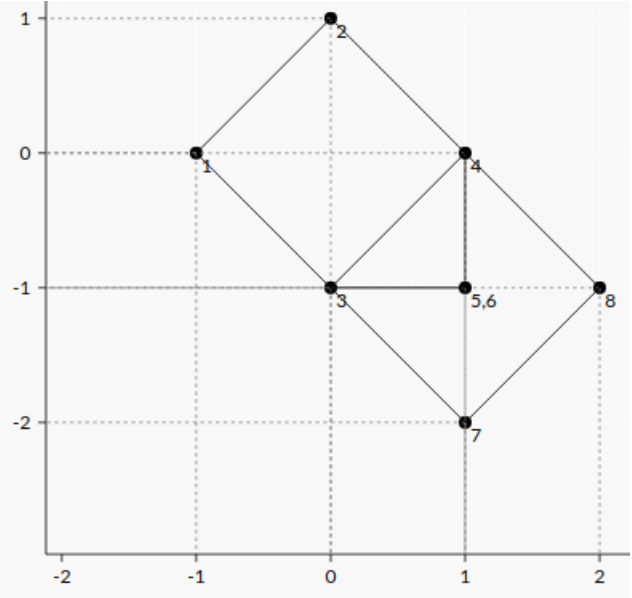시간 제한 메모리 제한 제출 정답 맞은 사람 정답 비율
1 초 256 MB 5 2 2 40.000%

문제

There are N points on the plane; the i-th point is at (Xi, Yi). There may be multiple points at the same location. Four of the points will be coloured black and three other points will be coloured white. The remaining N − 7 points will be uncoloured.

An oblong is a rectangle that is not a square.

An right triangle is a triangle where one of the interior angles is exactly ninety degrees.

Determine the number of ways to colour the points such that the four black points are the vertices of an oblong and the three white points are the vertices of a right triangle. Note that both shapes should have positive area.

입력

Line 1 contains one integer N (7 ≤ N ≤ 24).

Line 2 contains N integers X1, . . . , XN (−229 ≤ Xi ≤ 229).

Line 3 contains N integers Y1, . . . , YN (−229 ≤ Yi ≤ 229).

출력

Print one line with one integer, the number of ways to choose an oblong and a right triangle.

예제 입력 1

8
-1 0 0 1 1 1 1 2
0 1 -1 0 -1 -1 -2 -1

2

힌트

The only way to form an oblong is with points 1,2,7,8. Of the remaining four points there are two ways to form a right triangle from them.